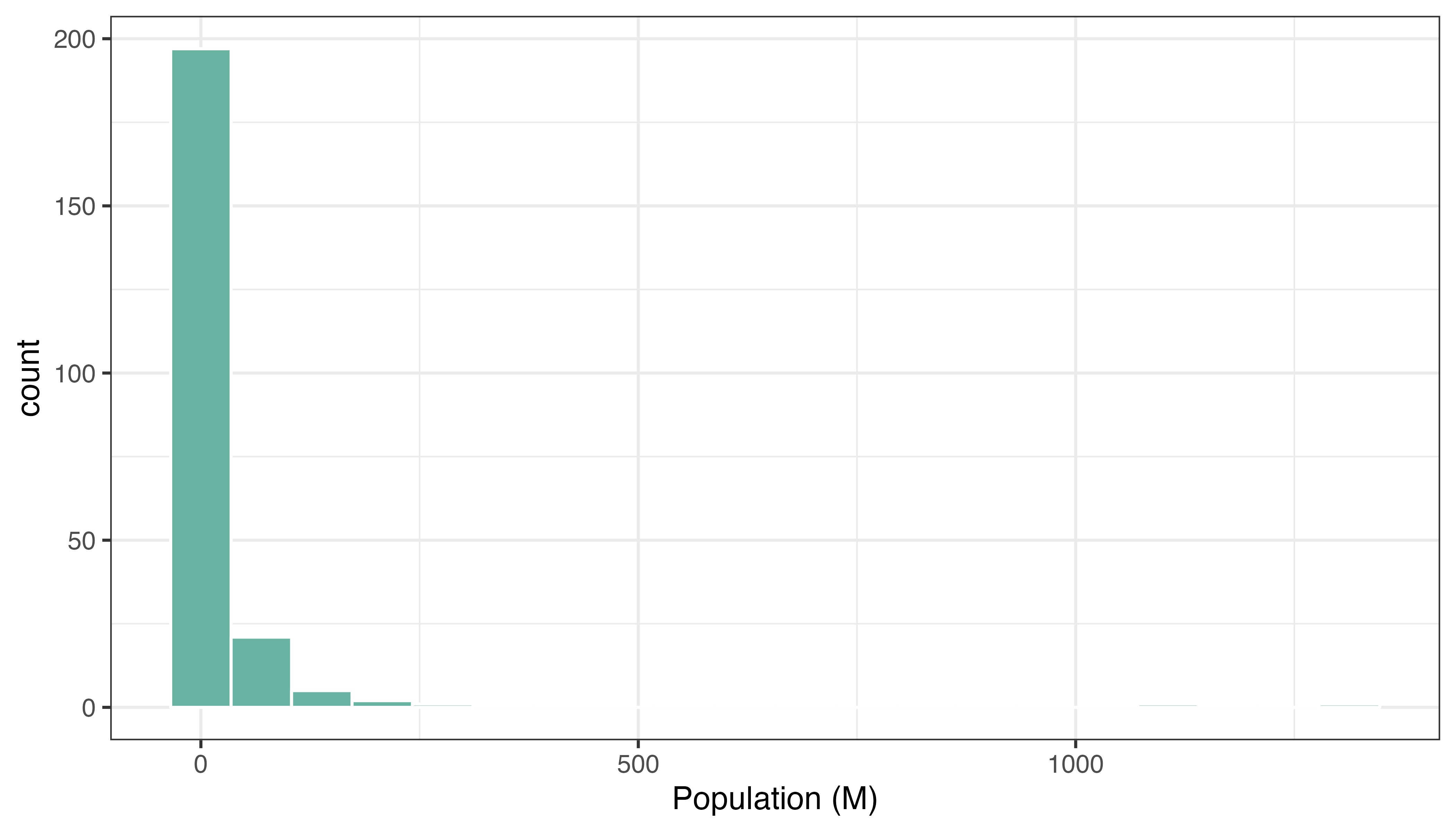# Interactive choropleth map with R and leaflet

This post explains how to build an interactive choropleth map with R and the `leaflet` package. A tooltip is available for each region on hover, and the map is zoomable.

`leaflet` is a R package allowing to build interactive maps. If you’re not familiar to it, have a look to this leaflet introduction. This post is a step-by-step tutorial leading to the following choropleth map.

Note: this step is described in detail here. Read it if you are not familiar with geospatial data management in R.

The region boundaries required to make maps are usually stored in geospatial objects. Those objects can come from shapefiles, geojson files or provided in a R package. See the background map section for possibilities.

This tutorial uses a geospatial object stored in a shape file available here. Start by downloading the file:

``````# Download the shapefile. (note that I store it in a folder called DATA. You have to change that if needed.)
# You now have it in your current working directory, have a look!

# Unzip this file. You can do it with R (as below), or clicking on the object you downloaded.
system("unzip DATA/world_shape_file.zip")
#  -- > You now have 4 files. One of these files is a .shp file! (TM_WORLD_BORDERS_SIMPL-0.3.shp)``````

``````# Read this shape file with the rgdal library.
library(rgdal)
dsn= paste0(getwd(),"/DATA/world_shape_file/") ,
layer="TM_WORLD_BORDERS_SIMPL-0.3",
verbose=FALSE
)

# Clean the data object
library(dplyr)
world_spdf@data\$POP2005[ which(world_spdf@data\$POP2005 == 0)] = NA
world_spdf@data\$POP2005 <- as.numeric(as.character(world_spdf@data\$POP2005)) / 1000000 %>% round(2)

# -- > Now you have a Spdf object (spatial polygon data frame). You can start doing maps!``````

# Default choropleth

It is now possible to draw a first choropleth map. Here are the main steps to follow:

• create a color palette thanks to the `colorNumeric()` function
• make the background map with `leaflet()`, `addTiles()` and `setView()`
• use `addPolygons()` to add the shape of all country, with a color representing the number of people in the country.

The resulting map is quite disapointing: China and India having very numerous population, all the variation between other countries gets hard to observe on the map.

``````# Library
library(leaflet)

# Create a color palette for the map:
mypalette <- colorNumeric( palette="viridis", domain=world_spdf@data\$POP2005, na.color="transparent")
mypalette(c(45,43))

# Basic choropleth with leaflet?
m <- leaflet(world_spdf) %>%
setView( lat=10, lng=0 , zoom=2) %>%
addPolygons( fillColor = ~mypalette(POP2005), stroke=FALSE )

m

# save the widget in a html file if needed.
# library(htmlwidgets)
# saveWidget(m, file=paste0( getwd(), "/HtmlWidget/choroplethLeaflet1.html"))``````

# Visualize the numeric variable

In a choropleth map, each region has a color that represents the value of a numeric variable (population here).

It is a good practice to check the distribution of this variable to understand what kind of color scale should be used. Using a histogram is often a good option for that:``````# load ggplot2
library(ggplot2)

# Distribution of the population per country?
world_spdf@data %>%
ggplot( aes(x=as.numeric(POP2005))) +
geom_histogram(bins=20, fill='#69b3a2', color='white') +
xlab("Population (M)") +
theme_bw()``````

# Change color scale

There are several ways to translate a numeric variable to a palette of color. Leaflet offers 3 options:

• quantile with `colorQuantile`
• numeric with `colorNumeric`
• bin with `colorBin`

Results can be very different and the best option usually depends on the distribution of your input data.

Quantile
Numeric
Bins
``````# Color by quantile
m <- leaflet(world_spdf)%>% addTiles()  %>% setView( lat=10, lng=0 , zoom=2) %>%
addPolygons( stroke = FALSE, fillOpacity = 0.5, smoothFactor = 0.5, color = ~colorQuantile("YlOrRd", POP2005)(POP2005) )
m

# Numeric palette
m <- leaflet(world_spdf)%>% addTiles()  %>% setView( lat=10, lng=0 , zoom=2) %>%
addPolygons( stroke = FALSE, fillOpacity = 0.5, smoothFactor = 0.5, color = ~colorNumeric("YlOrRd", POP2005)(POP2005) )
m

# Bin
m <- leaflet(world_spdf)%>% addTiles()  %>% setView( lat=10, lng=0 , zoom=2) %>%
addPolygons( stroke = FALSE, fillOpacity = 0.5, smoothFactor = 0.5, color = ~colorBin("YlOrRd", POP2005)(POP2005) )
m

# save the widget in a html file if needed.
# library(htmlwidgets)
# saveWidget(m, file=paste0( getwd(), "/HtmlWidget/choroplethLeaflet2.html"))``````

# Customizied leaflet choropleth map

In order to get a quality choropleth map, there are several improvements we need to apply:

• Add a legend with `addLegend()`
• Change the color scale: binning is a good option here since it avoids to put too much weight on China and India
• Add a tooltip with `labelOptions`. When you hover a specific region, a box appears with custom text. This is very handy to add additional information to your map.

Here is the result and the code:

``````# Create a color palette with handmade bins.
library(RColorBrewer)
mybins <- c(0,10,20,50,100,500,Inf)
mypalette <- colorBin( palette="YlOrBr", domain=world_spdf@data\$POP2005, na.color="transparent", bins=mybins)

# Prepare the text for tooltips:
mytext <- paste(
"Country: ", world_spdf@data\$NAME,"<br/>",
"Area: ", world_spdf@data\$AREA, "<br/>",
"Population: ", round(world_spdf@data\$POP2005, 2),
sep="") %>%
lapply(htmltools::HTML)

# Final Map
leaflet(world_spdf) %>%
setView( lat=10, lng=0 , zoom=2) %>%
fillColor = ~mypalette(POP2005),
stroke=TRUE,
fillOpacity = 0.9,
color="white",
weight=0.3,
label = mytext,
labelOptions = labelOptions(
style = list("font-weight" = "normal", padding = "3px 8px"),
textsize = "13px",
direction = "auto"
)
) %>%
addLegend( pal=mypalette, values=~POP2005, opacity=0.9, title = "Population (M)", position = "bottomleft" )

m

# save the widget in a html file if needed.
# library(htmlwidgets)
# saveWidget(m, file=paste0( getwd(), "/HtmlWidget/choroplethLeaflet5.html"))``````

Related chart types

## Contact

This document is a work by Yan Holtz. Any feedback is highly encouraged. You can fill an issue on Github, drop me a message on Twitter, or send an email pasting yan.holtz.data with gmail.com.